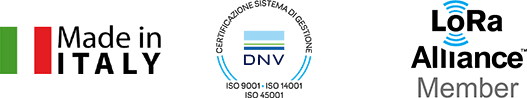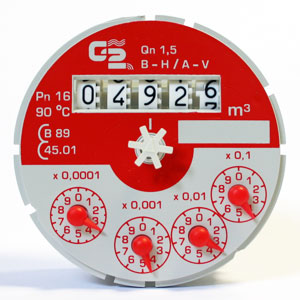# Instructions

### Instructions for a correct reading of the meter dialThe black digit rolls show the cubic meters (the black color identifies always the measuring unit, regardless the dimension of the rolls), on the other hand the red pointers or the red digit rolls show the submultiples of cubic meter.

In particular the red pointers at the middle of each small dial show the submultiples of the cubic meter: it is therefore possible to read also each liter (0,0001 cubic meter = 1 litre). Furthermore the small dial with x 0,0001 on it, has a double division to show a precision up to 1/20 part of the litre.

The dial in the picture shows digit rolls for 4926 cubic meter (the last roll on the right hand side is between numbers 6 and 7, therefore the reading will consider number 6, being the lower) The small dials on the lower part of the dial show:

dial x 0,1: value 4 (being just over the line of number 4) , therefore 0,4 cubic meter, i.e. 400 litres;

dial x 0,01: value 3 (being between 3 and 4), therefore 0,03 cubic meters, i.e. 30 lt

dial x 0,001: value 4 (being between 4 and 5), therefore 0,004 cubic meters, i.e. 4 litres

dial x 0,0001: value 3,5 (being exacly between 3 and 4), therefore 0,00035 cubic meters, i.e. 3,4 decilitre.

Adding up values we will obtain: 4.926,43435 cubic meters.

Usually the utilities supplying water, consider only the cubic meters, without reading the submultiples, after the comma.